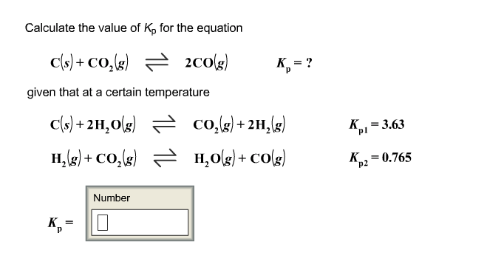# Problem: Calculate the value of Kp for the equation C(s) + CO2(g) ⇌ 2CO(g) Kp = ?given that at a certain temperatureC(s) + 2H2O(g) ⇌ CO2(g) + 2H2(g) Kp1 = 3.63H2(g) + CO2(g) ⇌ H2O(g) + CO(g) + Kp2 = 0.765Kp = ?

###### FREE Expert Solution
80% (194 ratings)###### Problem Details

Calculate the value of Kp for the equation

C(s) + CO2(g) ⇌ 2CO(g) Kp = ?
given that at a certain temperature
C(s) + 2H2O(g) ⇌ CO2(g) + 2H2(g) Kp1 = 3.63
H2(g) + CO2(g) ⇌ H2O(g) + CO(g) + Kp2 = 0.765

Kp = ?